# How to find the minimum and maximum values in Excel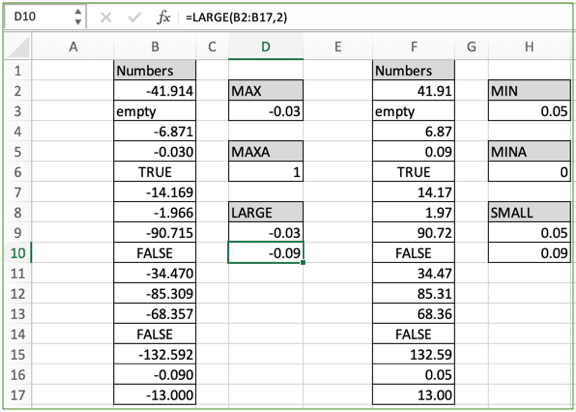In this article, we will learn How to find the minimum and maximum values in Excel.

Scenario:

There can be many scenarios where an excel user needs to find the minimum or maximum value from the given values. For example finding the minimum and maximum sales value in the year. Or finding the minimum and maximum spend on a grocery store. Excel provides you with such mathematical functions to get the result easily and conveniently. Here first we learn MAX, MAXA, LARGE and MAXIFS function.

MAX formulas in Excel

MAX function

MAX function ignores text, boolean (TRUE/FALSE) or any other non numeric values. The function takes the arguments as arrays as explained below.

MAX formula Excel

 =MAX(array)

MAXA function

MAXA function considers text as 0, boolean values TRUE as 1 and FALSE as 0 whereas numeric values are treated the same as MAX function. The MAXA function takes the arguments as arrays as explained below.

MAXA formula Excel

 =MAXA(array)

LARGE function

LARGE function takes the array and a value n as arguments and the function returns the nth largest or nth highest or nth max value.

LARGE formula Excel

 =LARGE(array,n)

array : list of numbers given as array references argument. Like values in A1 to A75 be referred as A1:A75

Example :

All of these might be confusing to understand. Let's understand how to use the function using an example. Here we have some values and we need to get the highest of all the values. Use the MAX formula so as to get the max value

Use the MAX formula:

 =MAX(B2:B17)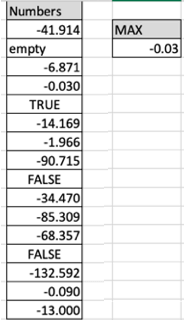The maximum value among these negative values is -0.03. Now we try the same array with the MAXA function as explained below.

Use the MAXA formula:

 =MAXA(B2:B17)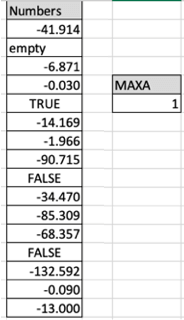As you know the highest value is -0.03 but as the function considers boolean values TRUE as 1 and FALSE 0. So it returns 1 as highest (B6) in B6 cells. Let's learn how to use Large function to get the nth max value in excel

LARGE function Example

To get the nth highest value from the list. To get the highest value, use the value of n as 1. To get the 2nd highest value, use the value of n as 2 and to get the smallest value using Large function use the value of n equal to length of array.

Use the formula:

 =LARGE(B2:B17,1)    # to get the max value

Use the formula:

 =LARGE(B2:B17,2)    # to get the 2nd max value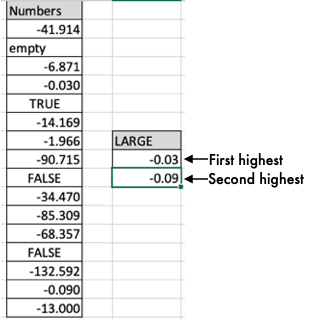As you can see the highest and the 2nd highest value evaluated using LARGE function in excel. Now let's get a hold on how to get the smallest values from the list.

MIN formulas in Excel

MIN function

MIN function ignores text, boolean (TRUE/FALSE) or any other non numeric values. The function takes the arguments as arrays as explained below.

MIN formula Excel

 =MIN(array)

MINA function

MINA function considers text as 0, boolean values TRUE as 1 and FALSE as 0 whereas numeric values are treated the same as MIN function. The MINA function takes the arguments as arrays as explained below.

MINA formula Excel

 =MINA(array)

SMALL function

SMALL function takes the array and a value n as arguments and the function returns the nth largest or nth highest or nth smallest or nth lowest value.

SMALL formula Excel

 =SMALL(array,n)

array : list of numbers given as array references argument. Like values in A1 to A75 be referred as A1:A75

Example :

All of these might be confusing to understand. Let's understand how to use the function using an example. Here we have some values and we need to get the highest of all the values. Use the MIN formula so as to get the minimum value

Use the MIN formula:

 =MIN(B2:B17)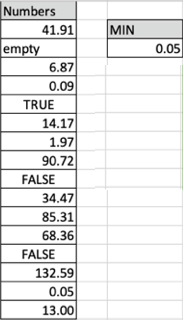The minimum value among these positive values is 0.05. Now we try the same array with the MINA function as explained below.

Use the MINA formula:

 =MINA(B2:B17)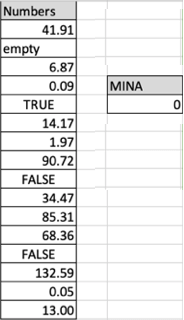As you know the lowest value is 0.05 but as the function considers boolean values TRUE as 1 and FALSE 0. So it returns 1 as lowest value in B10 cells. Let's learn how to use SMALL function to get the nth lowest value in excel

SMALL function Example

To get the nth lowest value from the list. To get the lowest value, use the value of n as 1. To get the 2nd lowest value, use the value of n as 2 and to get the largest value using a small function use the value of n equal to the length of the array.

Use the formula:

 =SMALL(B2:B17,1)    # to get the min value

Use the formula:

 =SMALL(B2:B17,2)    # to get the 2nd min value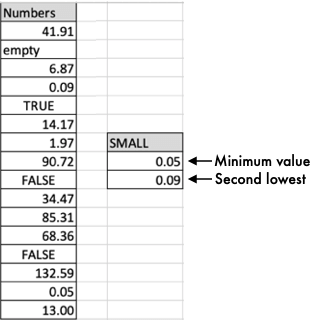As you can see the lowest and the 2nd lowest value evaluated using SMALL function in excel.

MAX/MIN with multiple criteria

Use the MAXIFS or MINIFS function in Excel to get the maximum value and minimum value satisfying the given multiple criteria. Learn how to use the MAXIFS function in Excel 365. Learn How to use the MINIFS function in Excel365. The above two functions are new and updated versions of Excel. find the Minimum value with IF criteria in Excel. Find maximum with if criteria in Excel for current and older version of excel.

Here are all the observational notes using the formula in Excel
Notes :

1. You can input manually or using array reference.
2. List of values can be given as array A1:A5000 or A:A (whole column).
3. All the above formulas consider empty cell and text values as 0.

Hope this article about How to find the minimum and maximum values in Excel is explanatory. Find more articles on statistical values and related Excel formulas here. If you liked our blogs, share it with your friends on Facebook. And also you can follow us on Twitter and Facebook. We would love to hear from you, do let us know how we can improve, complement or innovate our work and make it better for you. Write to us at info@exceltip.com.

Related Articles :

How to use the MAX function in Excel : returns the numerical largest value from the given array using the MAX function in Excel

How to use the MIN function in Excel : returns the numerical smallest value from the given array using the MIN function in Excel

How to use the LARGE function in Excel : returns the numerical largest nth value from the given array using the LARGE function in Excel.

Minimum value using IF function : Get the minimum value using the excel IF function and MIN function on array data.

Calculate max if condition match : Get the MAX value from the array having criteria.

How to Use Excel AGGREGATE Function : Use the AGGREGATE function to perform multiple operations with multiple criterias in excel.

COUNTIFS if either A or B or C Criteria : Count cells having multiple criteria match using the OR function.

How to use wildcards in excel : Count cells matching phrases using the wildcards in excel

Popular Articles :